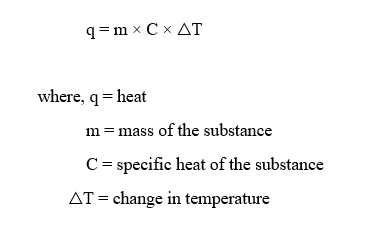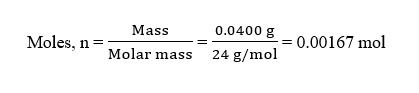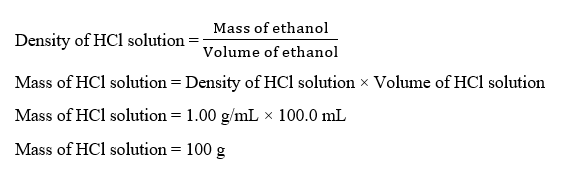# Suppose you place 0.0400 g of magnesium chips in a coffee-cup calorimeter and then add 100.0 mL of 1.00 M HCl. The reaction that occurs isMg(s) + 2 HCl(aq) → H2(g) + MgCl2(aq)The temperature of the solution increases from 21.66 °C (294.81 K) to 23.46 °C (296.61 K). What is the enthalpy change for the reaction per mole of Mg? Assume a specific heat capacity of the solution is 4.20 J/g∙K and the density of the HCl solution is 1.00 g/mL.kJ/mol

Question
27 views

Suppose you place 0.0400 g of magnesium chips in a coffee-cup calorimeter and then add 100.0 mL of 1.00 M HCl. The reaction that occurs is

Mg(s) + 2 HCl(aq) → H2(g) + MgCl2(aq)

The temperature of the solution increases from 21.66 °C (294.81 K) to 23.46 °C (296.61 K). What is the enthalpy change for the reaction per mole of Mg? Assume a specific heat capacity of the solution is 4.20 J/g∙K and the density of the HCl solution is 1.00 g/mL.

kJ/mol

check_circle

Step 1

The heat of a substance is given as :Step 2

Given,

Mass of Mg = 0.0400 g

Mass of HCl solution = 100.0 mL

Molarity of HCl solution = 1.00 M

Initial temperature, T1 = 294.81 K

Final temperature, T2 = 296.61 K

Specific heat capacity of solution, Csol = 4.20 J/g.K

Density of HCl solution = 1.00 g/mL

Step 3

Moles of Mg (n) can be calculated as :The mass of HCl solution can be calculated as :Here,

△T = T2 – T1 = 296.61 K – 294.81 K = 1.8 K

...

### Want to see the full answer?

See Solution

#### Want to see this answer and more?

Solutions are written by subject experts who are available 24/7. Questions are typically answered within 1 hour.*

See Solution
*Response times may vary by subject and question.
Tagged in